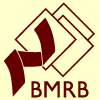# NMR Restraints Grid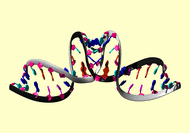Result table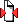(Save to zip file containing files for each block)

 image mrblock_id pdb_id cing stage position program type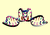476097 1bau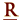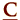cing 1-original 5 AMBER angle

```#flat angle constraint number   1
&rst  iat =  6,  6, 18, iresid = 1,
atnam(1)='N3   ',atnam(2)='H3  ',atnam(3)='O6   ',
r1 = 160.0, r2 = 170.0, r3 = 190.0, r4 = 210.0,
rk2 =20.001, rk3 =20.001, &end
# flat angle constraint number   2
&rst  iat =  6, 18, 18, iresid = 1,
atnam(1)='O2   ',atnam(2)='H1   ',atnam(3)='N1   ',
r1 = 160.0, r2 = 170.0, r3 = 190.0, r4 = 210.0,
rk2 =20.001, rk3 =20.001, &end

```

Please acknowledge these references in publications where the data from this site have been utilized.

Contact the webmaster for help, if required. Wednesday, October 20, 2021 11:52:31 PM GMT (wattos1)